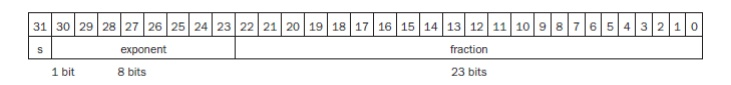Home | | Computer Architecture | Floating Point Operations

# Floating Point Operations

The scientific notation has a single digit to the left of the decimal point. A number in scientific notation that has no leading 0s is called a normalized number, which is the usual way to write it.

FLOATING POINT OPERATIONS

The scientific notation has a single digit to the left of the decimal point. A number in scientific notation that has no leading 0s is called a normalized number, which is the usual way to write it. Foating point - Computer arithmetic that represents numbers in which the binary point is not fixed. Floating-point numbers are usually a multiple of the size of a word.

The representation of a MIPS floating-point number is shown below, where s is the sign of the floating-point number (1 meaning negative), exponent is the value of the 8-bit exponent field (including the sign of the exponent), and fraction is the 23-bit number. This representation is called sign and magnitude, since the sign has a separate bit from the rest of the number.

A standard scientific notation for reals in normalized form offers three advantages. · It simplifies exchange of data that includes floating-point numbers; It simplifies the floating-point arithmetic algorithms to know that numbers will always be in this form;

·        It increases the accuracy of the numbers that can be stored in a word, since the unnecessary leading 0s are replaced by real digits to the right of the binary point.Fig. 2.14 Scientific notation

Step 1. To be able to add these numbers properly, align the decimal point of the number that has the smaller exponent. Hence, we need a form of the smaller number, 1.610ten x101, that matches the larger exponent. We obtain this by observing that there are multiple representations of an unnormalized floating-point number in scientific notation:

Step 1.1.610ten x101 = 0.1610ten x100 = 0.01610ten x101

Step 2. Next comes the addition of the significands: 9.999ten+ 0.016ten The sum is 10.015ten x101.

Step 3. This sum is not in normalized scientific notation, so we need to adjust it: 10.015ten x101 = 1.0015ten x102

Thus, after the addition we may have to shift the sum to put it into normalized form, adjusting the exponent appropriately.

Study Material, Lecturing Notes, Assignment, Reference, Wiki description explanation, brief detail
Computer Architecture : Arithmetic Operations : Floating Point Operations |